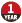# PAT (Basic Level) Practice 1002 写出这个数 python语言

2020-02-02 01:54 429 查看

​100
​​ 。

1234567890987654321123456789

yi san wu

python代码

```pinyin = {'0': 'ling', '1':'yi', '2':'er', '3':'san', '4':'si', '5':'wu', '6':'liu', '7':'qi', '8':'ba', '9':'jiu'}
a = input()
sum = 0
for i in a:
sum += eval(i)
sum = str(sum)
n = len(sum)
for i in range(n):
print(pinyin[sum[i]],end = '')
if i != n- 1 :
print(end = ' ')```
• 点赞
• 收藏
• 分享
• 文章举报Eumenides丶 发布了25 篇原创文章 · 获赞 0 · 访问量 996 私信 关注